GMAT  >  Test: Sale And Purchase- 1

# Test: Sale And Purchase- 1

Test Description

## 15 Questions MCQ Test Quantitative for GMAT | Test: Sale And Purchase- 1

Test: Sale And Purchase- 1 for GMAT 2023 is part of Quantitative for GMAT preparation. The Test: Sale And Purchase- 1 questions and answers have been prepared according to the GMAT exam syllabus.The Test: Sale And Purchase- 1 MCQs are made for GMAT 2023 Exam. Find important definitions, questions, notes, meanings, examples, exercises, MCQs and online tests for Test: Sale And Purchase- 1 below.
Solutions of Test: Sale And Purchase- 1 questions in English are available as part of our Quantitative for GMAT for GMAT & Test: Sale And Purchase- 1 solutions in Hindi for Quantitative for GMAT course. Download more important topics, notes, lectures and mock test series for GMAT Exam by signing up for free. Attempt Test: Sale And Purchase- 1 | 15 questions in 30 minutes | Mock test for GMAT preparation | Free important questions MCQ to study Quantitative for GMAT for GMAT Exam | Download free PDF with solutions
 1 Crore+ students have signed up on EduRev. Have you?
Test: Sale And Purchase- 1 - Question 1

### Product Y was sold after applying successive discounts of 20% and 30% on the list price (marked price). What is the list price of product Y if it was sold at \$112?

Test: Sale And Purchase- 1 - Question 2

### A bicycle is sold after applying successive discounts of 10% and 20% on the list price at Shop A. The same is sold at a flat discount of 30% on the list price at Shop B. If the list price of bicycle is \$200 for both Shop A and Shop B, what is the positive difference (if any) between the final prices of bicycles sold at Shop A and Shop B?

Test: Sale And Purchase- 1 - Question 3

### The marked price of an LCD television is 100% higher than the cost price. If it is sold at a price of \$810 after applying two successive discounts of 10% each on the marked price, what is the cost price (in dollars) of LCD television?

Test: Sale And Purchase- 1 - Question 4

The marked price of a garment is 100% higher than the cost price. If it is sold at a price of \$112 after applying successive discounts of 20% and 30% on the marked price, what is the profit (in dollars) obtained by selling the garment?

Test: Sale And Purchase- 1 - Question 5

The marked price of Brand A Milk is 50% higher than the cost price. If it is sold at a price of \$2.7 after applying a discount of 10% on the marked price, what is the profit % in selling Brand A milk?

Test: Sale And Purchase- 1 - Question 6

A certain motorcycle company manufactured and sold each of its 1,200 motorcycles during the last fiscal year. Did the company make a profit on the sales of motorcycles?

(1) For each motorcycle sold, the company’s revenue was \$10,000

(2) The company’s expenses comprise a fixed expense of \$2,000,000 per quarter and an additional \$3,500 for each motorcycle.

Detailed Solution for Test: Sale And Purchase- 1 - Question 6

Steps 1 & 2: Understand Question and Draw Inferences

The company manufactured and sold 1,200 motorcycles during its fiscal year. The question is whether it made a profit, so you need to know the revenue and expenses.

Step 3: Analyze Statement 1

(1)          For each motorcycle sold, the company’s revenue was \$10,000

Statement 1 tells you that the company’s revenue was \$12,000,000 during its fiscal year. However, without knowing its expenses, you don’t know whether the company made a profit.

Statement 1 is not sufficient.

Step 4: Analyze Statement 2

(2)          The company’s expenses comprise a fixed expense of \$2,000,000 per quarter and an additional \$3,500 for each motorcycle.

Statement 2 tells you that the company’s annual expenses were \$8,000,000 fixed plus \$4,200,000 (\$3,500 × 1,200), for a total of \$12,200,000. However, without knowing the revenue, you don’t know whether the company made a profit.

Statement 2 is not sufficient.

Step 5: Analyze Both Statements Together (if needed)

Taken together, if the revenues were \$12.0m and the expenses were \$12.2m, you know that the company did not make a profit.

With these, you are able to answer the question, even though the answer is no.

Test: Sale And Purchase- 1 - Question 7

Sam bought a television set from a store at a discount of 10%. He sold the set to a friend at a further discount of 10%. What is the discount his friend got on the original list price of the television set?

Test: Sale And Purchase- 1 - Question 8

A certain petroleum station purchased g gallons of petroleum for d dollars per gallon, and then it added e gallons of ethanol at f dollars per gallon. If this entire mixture were sold at h dollars per gallon, which of the following represents the gross profit per gallon on the sale of the mixture?

Detailed Solution for Test: Sale And Purchase- 1 - Question 8

Step 1: Question statement and Inferences

The station purchased g gallons of petroleum for d dollars per gallon, and then added e gallons of ethanol at f dollars per gallon.

The total volume of the mixture = (g + e) gallons

Step 2: Finding required values

The cost price of g gallons of petroleum = dg dollars

The cost price of e gallons of ethanol = ef dollars

So, the total cost price of the mixture = (dg + ef) dollars

The cost per gallon of the mixture =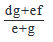Step 3: Calculating the final answer

Profit per gallon is given by Selling price per gallon – cost price per gallon.

We know that the selling price per gallon is h dollars, thus, profit is as shown: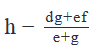Test: Sale And Purchase- 1 - Question 9

A certain electronics store purchased 500 tablets for \$150 each. If it sold some at a 20% markup and the rest at a 40% markup, how many were sold at a 40% markup?

(1) Had the store sold all the tablets at a markup of 30%, its gross profit would have been 6.25% lower.

(2) The gross profit from the 40% lot were three times the gross profit from the 20% lot.

Detailed Solution for Test: Sale And Purchase- 1 - Question 9

Steps 1 & 2: Understand Question and Draw Inferences

The electronics store marked up all of its 500 tablets. The question is how many were sold at a 40% markup? You can infer that the tablets were marked up either 20% or 40%, but no other percentage.

Let the number of tablets marked up at 20% be x.

Therefore, the number of tablets marked up at 40% will be 500 – x.

The selling price (SP) of and the profit made upon the sale of each 20% marked up and the 40% marked up tablet is calculated as below: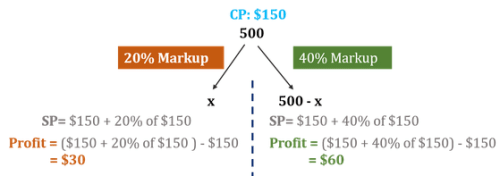We need to find the value of 500 – x. For this, we need to know the value of x.

We will now determine if Statements 1 and 2, alone or together, are sufficient to give us the value of x.

Step 3: Analyze Statement 1

(1)   Had the store sold all the tablets at a markup of 30%, its gross profit would have been 6.25% lower.

Gross profit in the scenario given in the question statement

= 30x + 60(500 – x)

= 30x + 30,000 – 60x

= 30,000 – 30x

Let’s now consider the scenario given in Statement 1:

SP = \$150 + 30% of \$150

Profit per tablet = SP – CP = (\$150 + 30% of \$150) -\$150 = 30% of \$150 = \$45

Gross profit upon the sale of 500 tablets = 45(500) = \$22,500

Per Statement 1,

22,500 = (30,000 – 30x) – 6.25% of (30,000 – 30x)

Thus, Statement 1 gives us a linear equation with only one unknown.

Therefore, upon solving this equation, we will be able to get a unique value of x.

Statement 1 alone is therefore sufficient.

Step 4: Analyze Statement 2

(2)  The gross profit from the 40% lot were three times the gross profit from the 20% lot.

Representing this mathematically,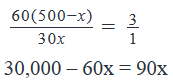Again, we get a linear equation with only one unknown. Upon solving this equation, we will be able to find a unique value of x

Statement 2 is sufficient.

Step 5: Analyze Both Statements Together (if needed)

You get a unique answer in steps 3 and 4, so this step is not required

Test: Sale And Purchase- 1 - Question 10

Jamie purchased three items from a budget store. She received discounts of 40% on the marked price of item A, 20% on the marked price of item B and 10% on the marked price of item C. How much money did she save, in percent terms, by shopping at the budget store?

(1) The marked price of item B is 75% of the marked price of item A and 200% more than the marked price of item C.

(2) The marked price of item A is 400% of the marked price of item C.

Detailed Solution for Test: Sale And Purchase- 1 - Question 10

Steps 1 & 2: Understand Question and Draw Inferences

Let the marked price of item A be a dollars,

The marked price of item B be b dollars, and

The marked price of item C be c dollars

So, had she not shopped at the budget store, she would have bought these three items at their marked price.

So, she would have spent a total of (a+b+c) dollars to buy these three items.

At the budget store,

Let the selling price of item A be a’

=> a’ = a – 40% of a  = 0.6a

Let the selling price of item B be b’

=> b’ = b – 20% of b = 0.8b

Let the selling price of item C be c’

=> c’ = c – 10% of c = 0.9 c

So, the money actually paid by Jamie for the three items = (0.6a + 0.8b + 0.9c)

Money saved by her by shopping at the budget store = (Money she would have otherwise spent to buy these 3 items) – (Money she spent at the budget store to buy these three items)

=> Money saved = (a + b + c) – (0.6a + 0.8b + 0.9c)

=> Money saved = 0.4a + 0.2b + 0.1c

% Moneysaved=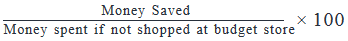% Moneysaved=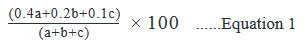Thus, in order to find the % Money saved, we need to know the values of a, b and c.

Step 3: Analyze Statement 1 independently

Statement 1 states that:

The marked price of item B is 75% of the marked price of item A and 200% more than the marked price of item C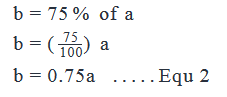Also,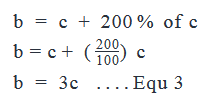Substituting Equation 3 in Equation 2:

3c = 0.75 a

⇒ c = 0.25a   ..... Equ 4

Substituting Equations 2 and 4 in Equation 1: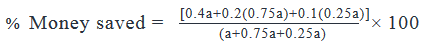We see that if we divide the numerator and denominator by a, we will get a tangible number. We don’t need to actually solve this expression, since this is a DS question and not a PS question. But we do know that we will be able to get a unique value for % Money Saved.

Therefore, Statement 1 alone is sufficient to arrive at a unique answer.

Step 4: Analyse Statement 2 independently

Statement 2 states that:

The marked price of item A is 400% of the marked price of item C

=>  a = 400% of c

=> a = 400/100 * c

=> a = 4c

However, Statement 2 gives us no idea of the value of b.

So, we will not be able to solve Equation 1 to determine a unique value of % Money Saved.

Therefore, Statement 2 is not sufficient.

Step 5: Analyse both statements together

This step is not required since we do get a unique solution in Step 3.

Test: Sale And Purchase- 1 - Question 11

In 2012, the marked price of an electric vehicle was \$50,000 and it was sold at a discount of n%. In early 2013, due to rise in fossil fuel prices, the demand for electric vehicles surged. Consequently, the price of the aforementioned electric vehicle was raised by m% over its earlier discounted price. What was the new selling price of the electric vehicle?

(1)  m=10

(2)  (100-n)(100+m)=2200

Detailed Solution for Test: Sale And Purchase- 1 - Question 11

Steps 1 & 2: Understand Question and Draw Inferences

We are given that the original marked price (say P) of an electric vehicle is \$50,000. In 2012, this product was being sold at a discount of n%.

P = \$50,000

So, the selling price Q of the product in 2012: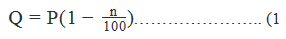In 2013, the demand for the electric vehicle increased and its prices was raised by m% over its discounted price in 2012. Let’s say its new selling price is R: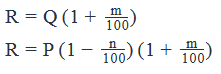By simplifying the above expression, we get: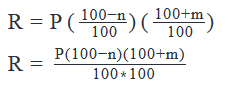By plugging in the value of P in the above equation,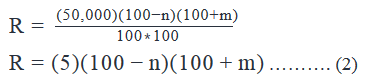So, we need to find the value of m and n to answer the question “What is the final price of the vehicle?”

Step 3: Analyze Statement 1

Statement I says: m = 10

This statement gives us the value of m only. We still don’t have the value of n.  So, we can’t determine the value of R.

Hence, statement I alone is not sufficient to answer the question: “What is the final price of the vehicle?”

Step 4: Analyze Statement 2

Statement 2 says: (100-n)(100+m)=2200

The expression given in this statement can directly be used in equation (2) to find the value of R.

So, statement II alone is sufficient to answer the question: “What is the final price of the vehicle?”

Step 5: Analyze Both Statements Together (if needed)

Since step 4 provides a unique answer to the question, we don’t need to perform this step.

Test: Sale And Purchase- 1 - Question 12

Stuart paid \$60 for purchasing a pair of identical chairs. He decided to sell the chairs at a price equal to the purchase price plus a markup that is 40 percent of the marked price, set by him. When he could not sell the chairs for one day, he discounted their marked price by 20 percent and quickly sold one chair. Worrying that he had underpriced the chairs, he raised the marked price of the remaining chair by 20 percent and sold it by the end of the day. What was his total profit or loss for the combined transactions?

Detailed Solution for Test: Sale And Purchase- 1 - Question 12

Step 1: Question statement and Inferences

Both chairs have the same cost price but different selling prices. We need to find the price at which each chair sells, in order to calculate the total profit made by selling the two chairs.

Step 2: Finding required values

Stuart purchased two chairs for \$60

So, Cost price of one chair, CP = 60/2 = \$30

Let the marked price of each chair be MP

MP = CP + Markup

Given: Markup = 40% of MP

So, MP = CP + 40% of MP

Or, 60% of MP = CP

60100MP=CPMP=10060×30=50

After 1 day, he discounted the marked price by 20%

So,new Marked Price=MP′=MP–20% of MP

=80 % of MP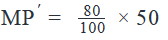MP′=40

He sold one chair at this price.

His profit on selling this chair = P1 = MP′ – CP

P1 = 40 – 30

P1 =\$ 10

For the unsold chair,

He raised the marked price by 20%

New Marked Price = MP′′=MP′+20 of MP′

MP′′ = 120 of MP′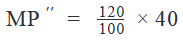MP′′=\$48

He sold the second chair at this price.

His profit on selling this chair

= P2 = MP′′–CP

P2 = 48 – 30

P2 = \$18

Step 3: Calculating the final answer

Total profit earned on the sale of the two chairs

= P1 + P2 = \$10 + \$18 = \$28

Looking at the answer choices, we see that Option C is correct

Test: Sale And Purchase- 1 - Question 13

A dealer bought a merchandise at a% less than its marked price and then sold at b% less that its marked price. What was the value of the discount offered to customers?

(1) a = 6%

(2) The dealer made a profit of 5%

Detailed Solution for Test: Sale And Purchase- 1 - Question 13

Steps 1 & 2: Understand Question and Draw Inferences

In this question, both CP and SP are expressed in terms of the marked price.

Let the marked price of the item be M

The cost price at which the merchant had bought the item was a % less than the marked price

=> CP = M – a % of M

=> CP = (1 - a/100) M

The selling price of the item was b % less than the marked price

=> SP = M – b % of M

=> SP = (1 - b/100) M

Discount offered to the customers = Marked Price – Selling Price

=> Discount = M - (1 - b/100) M

=> Discount = bM/100  . . . Equation 1

Thus, in order to determine the value of Discount, we need to know the values of b and M.

Step 3: Analyze Statement 1 independently

According to Statement 1,

a = 6%

However, this doesn’t give us any idea of the values of b and M.

So, Statement 1 is not sufficient.

Step 4: Analyze Statement 2 independently

As per Statement 2,

The dealer made a profit of 5%

Given: Profit = 5% of CP

But Profit = SP – CP

=> SP – CP = 5% of CP

=> SP = CP + 5% of CP

=> SP = 105% of CP

Substituting the values of SP and CP in the above expression: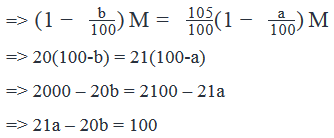However, we are not able to solve any further for the values of a and b. Moreover, we have no idea of the value of M.

Therefore, we cannot find the value of Discount using Statement 2.

Therefore, Statement 2 too is not insufficient

Step 5: Analyze Both Statements Together (if needed)

From Statement 1,

a = 6

From Statement 2,

21a – 20b = 100

Using both these Statements together, we will be able to find the value of b.

But we will still not know the value of M.

Therefore, we cannot find the value of Discount even by analysing both the statements together.

Therefore, the correct answer is E.

Note:  If the question had asked about discount percentage instead of the value of discount, we would have been able to answer the question by analysing both statements together and the answer would have been C.

This is because,

Discount percentage = b %

Test: Sale And Purchase- 1 - Question 14

A furniture dealer purchased 10 sofas for \$500 each. If he sells all the sofas for no more than \$600 each, what is the lowest price that he can sell any of the sofas to earn an average gross profit of \$90 per sofa?

Detailed Solution for Test: Sale And Purchase- 1 - Question 14

Step 1: Question statement and Inferences

The furniture dealer purchased 10 sofas for \$500 each. An average gross profit of \$90 per sofa means the average sale price is \$590, and no sofa can be sold for more than \$600.

Step 2: Finding required values

To find the lowest price of one sofa, assume the other 9 sofas were sold at their highest prices, which is \$600 each, for an average of \$590.

Step 3: Calculating the final answer

Let the price of the lowest price sofa be \$x. Then we can set up the equation like this:

9(600)+x10=590

And solve for x:

5,400+x=5,900

x = 500

Test: Sale And Purchase- 1 - Question 15

If a material is sold for Rs. 34.80,Rs. 34.80, there is a loss of 25%.25%. Find out the cost price of the material?

Detailed Solution for Test: Sale And Purchase- 1 - Question 15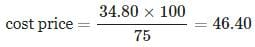## Quantitative for GMAT

88 videos|99 docs|165 tests
 Use Code STAYHOME200 and get INR 200 additional OFF Use Coupon Code
Information about Test: Sale And Purchase- 1 Page
In this test you can find the Exam questions for Test: Sale And Purchase- 1 solved & explained in the simplest way possible. Besides giving Questions and answers for Test: Sale And Purchase- 1, EduRev gives you an ample number of Online tests for practice

## Quantitative for GMAT

88 videos|99 docs|165 tests